# Spacecraft Attitude Determination Using Global Positioning Satellite Signals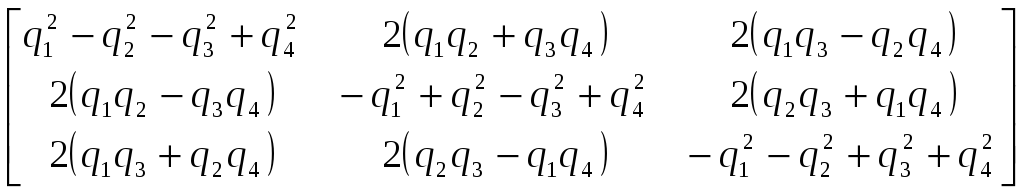(12) Taking the transpose of this matrix will provide the transformation matrix from the body frame to the local orbital (Steyn). Adding the transformed receiver vectors to the spacecraft’s and subtracting this vector from the GPS position vector yields the vector from the receiver to the GPS satellite. Taking the magnitude of this vector, the fractional part can be separated, Gaussian noise can be added, and it is ready to be used in the simulation. For simplicity, only receivers three through five are used with the two GPS satellites. To determine the integer phase differences for each of the baselines, all possible orientations for each baseline are calculated. These are compared and the orientation that matches across all three baselines is the correct orientation (with noise, the orientations that are within a given tolerance of each other, 0.1 wavelengths in this case, are assumed to be the same. The GN and GNLM methods follow the following algorithm: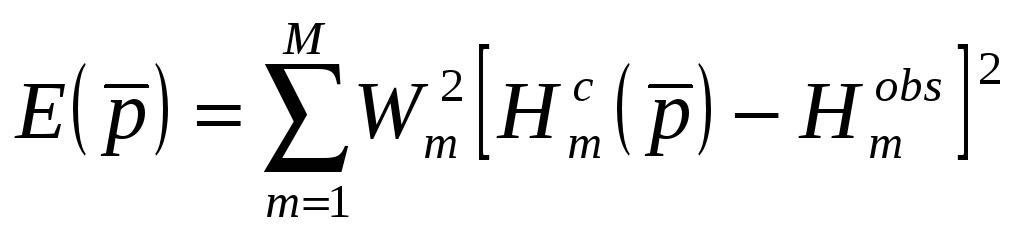(13) For which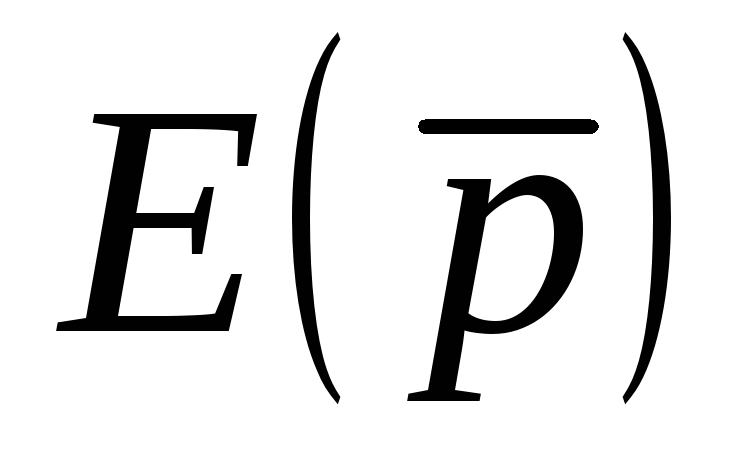is the error for the vector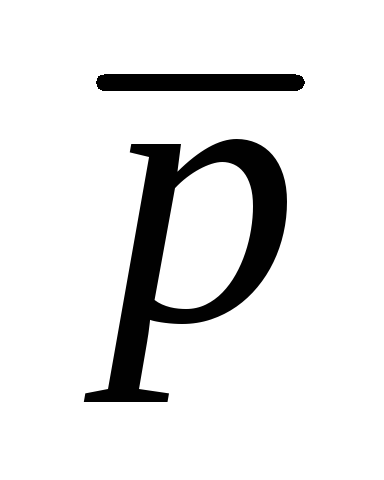the elements of which are being estimated,is the weighting matrix,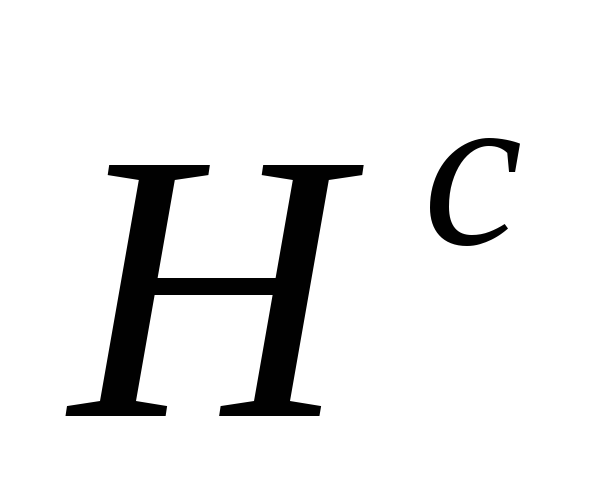is the calculated head, and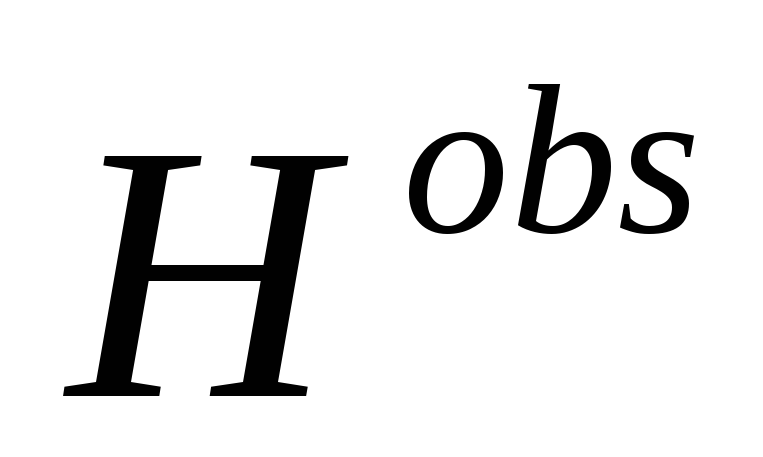is the measurement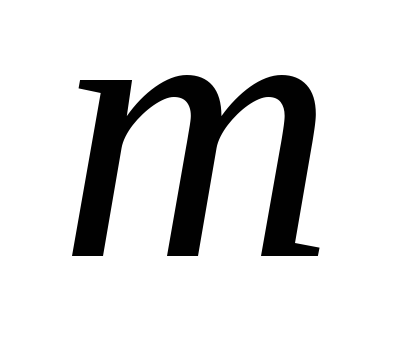(Gauss-Newton-Levenberg-Marquardt Method). Since it is a transformation matrix that needs to be estimated, not a vector, the vectorfor this case is defined as: Download 1.81 Mb.Share with your friends: